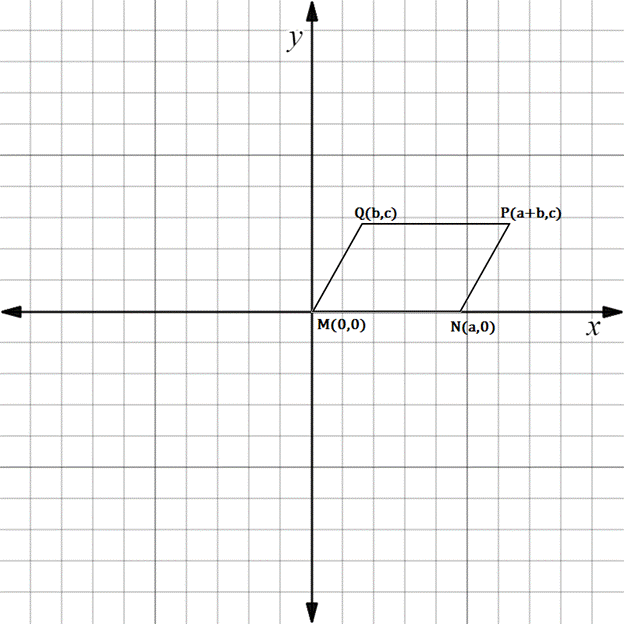Chapter 10.3, Problem 7EElementary Geometry For College St...

7th Edition
Alexander + 2 others
ISBN: 9781337614085

Solutions

Chapter
SectionElementary Geometry For College St...

7th Edition
Alexander + 2 others
ISBN: 9781337614085
Textbook Problem

In Exercises 5 to 10, the real numbers a, b, c, and d are positive.Consider the quadrilateral with vertices at M 0 ,   0 , N a ,   0 , P a + b ,   c , and Q b ,   c . Explain why M N P Q is a parallelogram.To determine

To explain:

MNPQ is a parallelogram.

Explanation

The given diagram is shown below.

To prove MNPQ is a parallelogram, we have to prove the following statement.

Both pairs of opposite sides are parallel.

MN is parallel to QP.

That is slope of MN is equal to slope of PQ.

Also, QM is parallel to PN.

That is slope of QM is equal to slope of PN.

Find the slope of MN.

x1,y1=M0, 0

x2,y2=Na, 0

Formula for slope joining the line segment.

Slope is denoted by m.

m=y2-y1x2-x1

Here x1x2

Substituting the x and y co-ordinates.

mMN=0-0a-0

mMN=0

Then, find the slope of QP.

x1,y1=Qb, c

x2,y2=Pa+b, c

Substituting the x and y co-ordinates.

mQP=c-ca+b-b

mQP=0a

mQP=0

mMN=mQP

Hence, MN and QP are parallel

Still sussing out bartleby?

Check out a sample textbook solution.

See a sample solution

The Solution to Your Study Problems

Bartleby provides explanations to thousands of textbook problems written by our experts, many with advanced degrees!

Get Started

4. Find the standard deviation of the data: 10, 12, 12, 8, 30, 12, 8, 10, 5, 23

Mathematical Applications for the Management, Life, and Social Sciences

State the Midpoint Rule.

Single Variable Calculus: Early Transcendentals

Multiply. (sin4)2

Trigonometry (MindTap Course List)